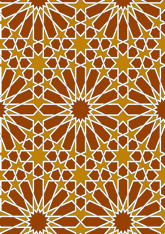data209/BON11

## Geometry

• The symmetry group of the tiling is 2*22 (cmm).
• All the internal angles of the constituent polygons are a multiple of 22.5°.
• Contains one regular 8-pointed star polygon with vertex angle of 45°.
• Contains one regular 16-pointed star polygon with vertex angle of 45°.
• There are 7 non-regular reflective tiles (including one kite).
• The tiling satisfies the interlace condition and has no finite interlace and two infinite interlaces with straight cross-overs.
• The tiling is edge-to-edge.
• As drawn, contains about 503 polygons.

## References

Publications referenced: